# 在物联网设备中运行 TensorFlow

## 概要

Google 关于 AI 的框架 TensorFlow 近几年来，也逐渐演化出来一个新的分支，嵌入式系统与机器学习。经过 TensorFlow Lite 的发展，在低功耗的微处理器领域，又形成了另外一个解决方案，即 TensroFlow Lite Micro（以下简称 TFLM），主要用于碎片化领域相当严重的嵌入式领域，针对跨平台操作的通用性，以及资源限制的场景。

• 2015 年 11 月，推出了 TensorFlow 0.1 版本。

• 2017 年 2 月，推出 TensorFlow 1.0 版本。

• 2018 年 5 月，推出 TensorFlow Lite。

• 2019 年 5 月，推出 TensorFlow Lite Micro。

## TensorFlow Lite 硬件

1. Edge TPU

Edge TUP 为 Google 专门设计的一款的 ASIC， 旨在运行边缘端的推理。

• 端到端的 AI 基础架构，协助客户部署端侧的 AI 解决方案。

• 体型小，功耗低，但是性能出色。

• 将硬件，软件，AI 算法结合在一起的解决方案。

• 众多工业使用场景。

Edge TPU 是端侧的 AI 解决方案。Edge TPU 对 CPU、GPU、FPGA 以及其他在边缘运行 AI 的 ASIC 解决方案构成了有益的补充。

_ Edge（设备/节点/网关/服务） Cloud

ML 框架 TensorFlow Lite, NN API TensorFlow, scikit-learn, XGBoost, Keras

Edge TPU 的硬件性能如下：用于加速 AI 推理的专用芯片，以 8 位整理的格式运算，硬件接口支持 PCIE 和 USB。

2. Coral

Coral 是一个完整的工具包，可以使用本地 AI 构建产品。它的设备端推理功能能够方便的构建高效、私密、快速和离线的产品。所有的硬件都完全支持 TensorFlow Lite 框架。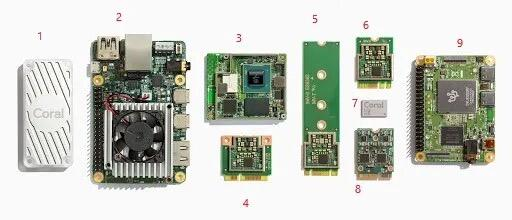device

1. Coral 的 USB 加速器，可以做为 Windows，Linux 和 Macos 的配件来使用，支持 TensorFlow Lite 推理。

2. Coral 开发板：支持的操作系统为 Mendel Linux（Debian 的衍生版本）。

3. System-on-Module (SoM)，可以理解为核心板。

4. 基于 Mini PCIE 接口的加速器，仅支持主机为 Debian Linux 系统。

5. 基于 M.2 的 A+E Key 加速器。

6. 基于 M.2 的 B+M Key 加速器。

7. 支持 Edge TPU 的芯片模块。

8. 支持双 Edge TPU 的 E Key 的加速器。

9. Coral 开发板，即 2 号板的迷你版。

• A 支持 PCIE (x2) 和 USB 接口

• E 支持 PCIE (x2) 和 USB 接口

• B 支持 SATA 接口

• M 支持 PCIE (x2/4) 接口

A+E 表示 A 和 E 都支持。这些信息有些绕，大概知道是 PCIE 和 USB 两种接口都支持就差不多了。

• ASUS AI Accelerator PCIe Card， 以 PCIe 加速卡的形式提供 Edge TPU 支持，最高支持 8 个 Coral M.2 加速卡。

• ASUS Tinker Edge T：以开发板的形式提供 Edg TPU 支持，基于 Coral SoM 板进行扩展。

Coral 硬件支持的算子如下：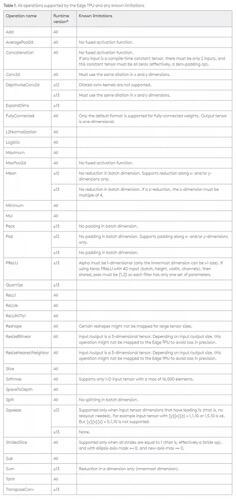coral operation

## TensorFlow Lite 软件

TensorFlow Lite 模型的整个编译流程如下：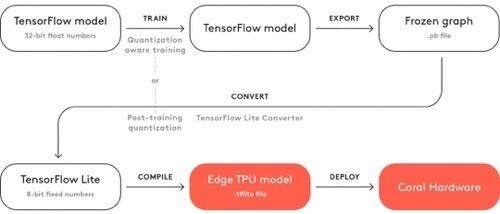1. 特性

• 缩短延迟：数据无需往返服务器

• 保护隐私：任何数据都不会离开设备

• 减少连接/降低功耗：不需要互联网连接

2. 优化方向

• 针对设备端机器学习进行了解释器的调整，对设备端的一组核心操作符进行了优化，生成较小的二进制。所支持的算子详见 这里

• 支持多种平台（涵盖 Android 和 iOS 设备、嵌入式 Linux 和微控制器），并利用平台 API 执行加速推断。

• 提供多种语言的 API，包括 Java、Swift、Objective-C、C++和 Python。

• 高性能，在支持的设备上执行硬件加速，其中对内核，以及激活函数都做了硬件层面的优化。

• 模型优化工具（包括量化），可在不影响准确率的情况下减小模型并提高其性能。

• 高效的模型格式，在大小和可移植性方面优化了 FlatBuffer。

3. 转换器

TensorFlow Lite 转换器执行流程如下：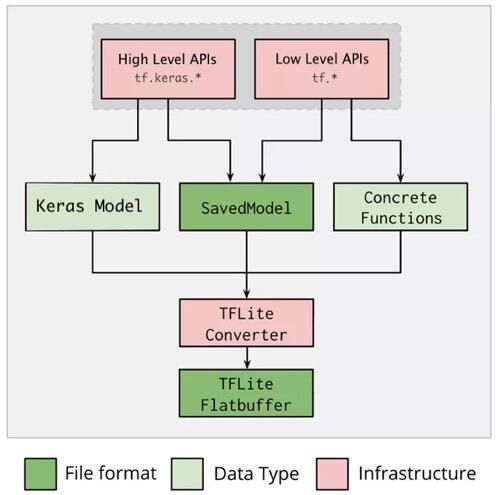• 命令行：仅支持基本模型转换。

SavedModel 转成 TensorFlow Lite 的代码如下：

``````# (输入参数为SavedModel的路径)
converter = tf.lite.TFLiteConverter.from_saved_model(saved_model_dir)
tflite_model = converter.convert()

# (生成tflite模型文件)
with open('model.tflite', 'wb') as f:
f.write(tflite_model)
``````

Keras 模型转成 TensorFlow Lite 模型

``````import tensorflow as tf

# (运用高阶的tf.keras API创建一个模型)
model = tf.keras.models.Sequential([
tf.keras.layers.Dense(units=1, input_shape=),
tf.keras.layers.Dense(units=16, activation='relu'),
tf.keras.layers.Dense(units=1)
])
model.compile(optimizer='sgd', loss='mean_squared_error') # compile the model
model.fit(x=[-1, 0, 1], y=[-3, -1, 1], epochs=5) # train the model

# (下一行代码可以把Keras保存为SavedModel，仅做功能提示)
# tf.saved_model.save(model, "saved_model_keras_dir")

# (转换模型)
converter = tf.lite.TFLiteConverter.from_keras_model(model)
tflite_model = converter.convert()

# (保存模型)
with open('model.tflite', 'wb') as f:
f.write(tflite_model)
``````

``````# 转换为SavedModel
tflite_convert \
--saved_model_dir=/tmp/mobilenet_saved_model \
--output_file=/tmp/mobilenet.tflite
``````
``````# 转换为Keras的H5模型
tflite_convert \
--keras_model_file=/tmp/mobilenet_keras_model.h5 \
--output_file=/tmp/mobilenet.tflite
``````

``````import tensorflow as tf

# (用tf.* API来创建一个模型)
class Squared(tf.Module):
@tf.function
def __call__(self, x):
return tf.square(x)
model = Squared()
# (运行模型)
result = Squared(5.0) # This prints "25.0"
# (产生SavedModel)
tf.saved_model.save(model, "saved_model_tf_dir")
concrete_func = model.__call__.get_concrete_function()

# (转换模型)
converter = tf.lite.TFLiteConverter.from_concrete_functions([concrete_func])
tflite_model = converter.convert()

# (保存模型)
with open('model.tflite', 'wb') as f:
f.write(tflite_model)
``````

• 算子支持 TensorFlow，但是不支持 TensorFlow Lite：如果模型有尺寸限制的话，需要直接创建 TensorFlow Lite 操作符，否则直接在TensorFlow Lite模型中直接使用 TensorFlow 操作符即可。

• 算子不支持 TensorFlow，同时也不支持 TensorFlow Lite，也就意味着，需要在 TensorFlow Lite 中自定义算子了。

``````import tensorflow as tf

#(以y=sin(x)为例自定义算子)
def TFLiteAwesomeCustomOp(x):
return tf.sin(x, name="TFLiteAwesomeCustomOp")

# (自定义算子描述输入输出描述)
custom_opdef = """name: 'TFLiteAwesomeCustomOp' input_arg:
{ name: 'In' type: DT_FLOAT } output_arg: { name: 'Out' type: DT_FLOAT }
attr : { name: 'a1' type: 'float'} attr : { name: 'a2' type: 'list(float)'}"""

# (在调用转换器相关的API之前，注册自定义的opdefs)
tf.lite.python.convert.register_custom_opdefs([custom_opdef])

converter = tf.lite.TFLiteConverter.from_saved_model(...)
converter.allow_custom_ops = True

``````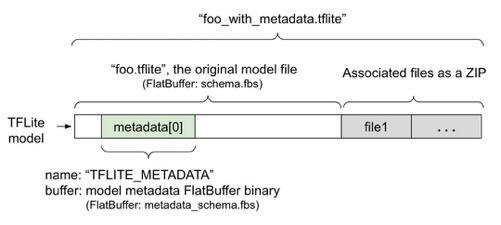``````# (转换为SavedModel)
tflite_convert \
--saved_model_dir=/tmp/mobilenet_saved_model \
--output_file=/tmp/mobilenet.tflite
``````
``````# (转换为Keras的H5模型)
tflite_convert \
--keras_model_file=/tmp/mobilenet_keras_model.h5 \
--output_file=/tmp/mobilenet.tflite
``````

4. 量化

TensorFlow Lite 的一个关键技术是量化，把浮点数转换为 int8 类型的整数，用于压缩模型，并且在推理阶段获得速度上的提升。量化一般是在模型转换阶段进行的，也就是在模型完成训练之后才进行的量化。

16 位浮点量化 原始尺寸 1/2，GPU 加速 CPU，GPU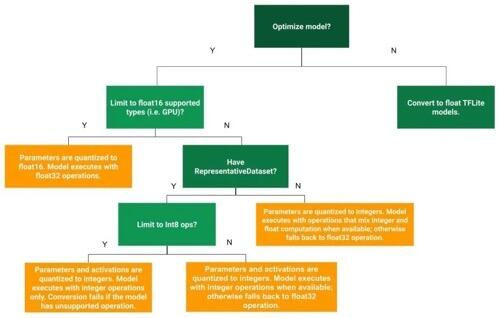``````# (动态范围量化，只是在生成模型时，把浮点转为8位精度的整形，但是在推理阶段，仍然从8位精度转换成浮点，并且使用硬件的浮点计算单元完成计算，输出仍然用浮点存储。)
import tensorflow as tf
converter = tf.lite.TFLiteConverter.from_saved_model(saved_model_dir)
converter.optimizations = [tf.lite.Optimize.DEFAULT]
tflite_quant_model = converter.convert()
``````

``````# （产生数据)
def representative_dataset():
for data in tf.data.Dataset.from_tensor_slices((images)).batch(1).take(100):
yield [tf.dtypes.cast(data, tf.float32)]

# (量化数据)
import tensorflow as tf
converter = tf.lite.TFLiteConverter.from_saved_model(saved_model_dir)
converter.optimizations = [tf.lite.Optimize.DEFAULT]
converter.representative_dataset = representative_dataset
tflite_quant_model = converter.convert()

# (有可能导致输出结果仍然是浮点数，会与Edge TPU，以及8位的微处理器不兼容。如果要确保输入和输出都与上述的硬件兼容的话，需要采用以下的代码：)
import tensorflow as tf
converter = tf.lite.TFLiteConverter.from_saved_model(saved_model_dir)
converter.optimizations = [tf.lite.Optimize.DEFAULT]
converter.representative_dataset = representative_dataset
converter.target_spec.supported_ops = [tf.lite.OpsSet.TFLITE_BUILTINS_INT8]
converter.inference_input_type = tf.int8  # or tf.uint8
converter.inference_output_type = tf.int8  # or tf.uint8
tflite_quant_model = converter.convert()
``````

16 位浮点量化

``````import tensorflow as tf
converter = tf.lite.TFLiteConverter.from_saved_model(saved_model_dir)
converter.optimizations = [tf.lite.Optimize.DEFAULT]
converter.target_spec.supported_types = [tf.float16]
tflite_quant_model = converter.convert()
``````

16 位浮点量化，也会带来一些副作用

• 优点：减少模型尺寸，精度损失最小，并且直接对 16 位的浮点进行运算。

• 缺点：比定点的延迟多，在没有浮点运算单元的设备上会进行量化的逆操作。

5. 解释器

• 将模型加载到内存中。

• 基于现有模型构建一个解释器。

• 设置输入张量值。（如果不需要预定义的大小，可以选择调整输入张量的大小。）

• 调用推理。

• 读取输出张量值。

``````class FlatBufferModel {
// 基于文件加载模型，如果创建失败，则返回nullptr
static std::unique_ptr<FlatBufferModel> BuildFromFile(
const char* filename,
ErrorReporter* error_reporter);

// 基于预加载的 flatbuffer 创建模型。 调用函数保留缓冲区的所有权并且保持它的活动直到返回的对象被销毁。 失败时返回 nullptr。
static std::unique_ptr<FlatBufferModel> BuildFromBuffer(
const char* buffer,
size_t buffer_size,
ErrorReporter* error_reporter);

// 备注： 如果 TensorFlow Lite 检测到Android NNAPI的存在 ，它会自动尝试使用共享内存来存储FlatBufferModel.
};
``````

``````// 注意： 不能在并发线程中调用解释器。

// 加载模型
std::unique_ptr<tflite::FlatBufferModel> model =
tflite::FlatBufferModel::BuildFromFile(filename);

// 创建解释器
tflite::ops::builtin::BuiltinOpResolver resolver;
std::unique_ptr<tflite::Interpreter> interpreter;
tflite::InterpreterBuilder(*model, resolver)(&interpreter);

// 如果需要的话，重定义输入张量
// 一旦调整完张量大小，就必须调用AllocateTensors来触发输入和输出张量的内存分配。
interpreter->AllocateTensors();

// 张量由整数表示，以避免与字符器进行比较
// 填充`input`.
float* input = interpreter->typed_input_tensor<float>(0);

interpreter->Invoke();

float* output = interpreter->typed_output_tensor<float>(0);
``````

## Coral 上运行

TensorFlow Lite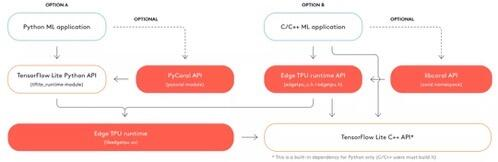• 基于 Python 或 C++在 Edge TPU 进行模型部署

• 在多个 Edge TPU 运行多个模型

• 在多 Edge TPU 的流水线上运行单个模型

• 也可以在自己的架构上编译并运行 libedgetpu

``````### 利用pycoral库运行推理
import os
import pathlib
from pycoral.utils import edgetpu
from pycoral.utils import dataset
from PIL import Image

# 导入TensorFlow Lite库，标签以及要识别的图片
script_dir = pathlib.Path(__file__).parent.absolute()
model_file = os.path.join(script_dir, 'mobilenet_v2_1.0_224_quant_edgetpu.tflite')
label_file = os.path.join(script_dir, 'imagenet_labels.txt')
image_file = os.path.join(script_dir, 'parrot.jpg')

# 初始化TensorFlow解释器
interpreter = edgetpu.make_interpreter(model_file)
interpreter.allocate_tensors()

# 重新定义图片尺寸
size = common.input_size(interpreter)
image = Image.open(image_file).convert('RGB').resize(size, Image.ANTIALIAS)

# 运行推理
common.set_input(interpreter, image)
interpreter.invoke()
classes = classify.get_classes(interpreter, top_k=1)

# 打印结果
``````

C++代码完成相同的任务

``````int main(int argc, char* argv[]) {
absl::ParseCommandLine(argc, argv);

// 加载模型
auto edgetpu_context = coral::ContainsEdgeTpuCustomOp(*model)
? coral::GetEdgeTpuContextOrDie()
: nullptr;
auto interpreter = coral::MakeEdgeTpuInterpreterOrDie(*model, edgetpu_context.get());
CHECK_EQ(interpreter->AllocateTensors(), kTfLiteOk);

// 读取图片到输出张量
auto input = coral::MutableTensorData<char>(*interpreter->input_tensor(0));
CHECK_EQ(interpreter->Invoke(), kTfLiteOk);

// 读取标签

for (auto result : coral::GetClassificationResults(*interpreter, 0.0f, /*top_k=*/3)) {
std::cout << "---------------------------" << std::endl;
std::cout << labels[result.id] << std::endl;
std::cout << "Score: " << result.score << std::endl;
}
return 0;
}
``````

``````# USB接口的Edge TPU设备
interpreter_usb1 = make_interpreter(model_1_path, device='usb:0')
# PCIE接口的Edge TPU设备
interpreter_pcie1 = make_interpreter(model_2_path, device='pci:0')
``````

1. 在 Edge TPU 运行 Model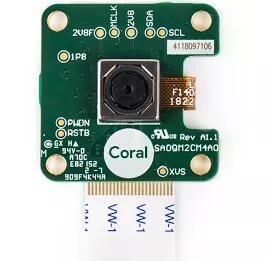camera

coral board

• 供电接口与数据线接口的区分，两个接口都是用的 type-c 接口。

• 供电电压 2v~3v，小于 2V 将无法正常启动开发板。

• 如果有显示需求，请外接 HDMI。

``````#安装开发工具
\$ pip3 install --user mendel-development-tool
#获取设备网址(网址为USB转网口产生的内部IP,不用关注)
\$ mdt devices
device-name                (192.168.100.2)

#下载源码,包含两个文件夹
#classifier-start为初始化代码
#classifier-end为最后结果的代码
``````edge tpu

``````#将模型及相关库拷贝到Edge TPU上
\$ cd classifier-start/
\$ mdt exec mkdir /home/mendel/lib
\$ mdt push lib/* /home/mendel/lib

\$ mdt push models/* /home/mendel
\$ mdt push main.py /home/mendel

# 获取设备终端，查看已拷贝内容
\$ mdt shell
...
[email protected]:~\$ ls
imagenet_labels.txt  inception_v2_224_quant.tflite  lib  main.py

# 运行模型
[email protected]:~\$ python3 main.py \
--model=inception_v2_224_quant.tflite \
--labels=imagenet_labels.txt
``````

``````# main.py
# 初始化推理引擎
from edgetpu.classification.engine import ClassificationEngine

def init_engine(model):
"""返回Edge TPU当前模型对像"""
return ClassificationEngine(model)

# 确定张量形状
def input_size(engine):
"""返回模型的输入大小"""
_, h, w, _ = engine.get_input_tensor_shape()
return w, h

# 分析推理
def inference_time(engine):
"""返回推理时间"""
return engine.get_inference_time()

# 分类
def classify_image(tensor, engine, labels):
"""返回推理时间及推理结果，推理结果包含标签索引以及置信度得分这两个参数"""
results = engine.ClassifyWithInputTensor(
tensor, threshold=0.1, top_k=3)
return [(labels[i], score) for i, score in results]
``````

``````# 添加源，并且安装相关软件

\$ echo "deb https://packages.cloud.google.com/apt coral-edgetpu-stable main" | sudo tee /etc/apt/sources.list.d/coral-edgetpu.list

\$ sudo apt-get update

\$ sudo apt-get install edgetpu-compiler

# 优化模型
\$ edgetpu_compiler model_A.tflite model_B.tflite
``````
``````# 更新后的脚本部署到设备
\$ mdt push main.py /home/mendel

#重新运行脚本
[email protected]:~\$ python3 main.py \
--model=inception_v2_224_quant.tflite \
--labels=imagenet_labels.txt

# 利用摄像头识别图片之后的结果
Inference time: 655.08 ms (1.53 fps)                goldfish, Carassius auratus (0.96)
``````

Edge TPU 的另一个优化方向是，总共有 8M 的 SRAM， 它可以用一段 SRAM 来缓存模型的参数，这样如果模型参数不变的化，第一次运行较慢，后面运行速度很快，缓存模型参数的流程如下：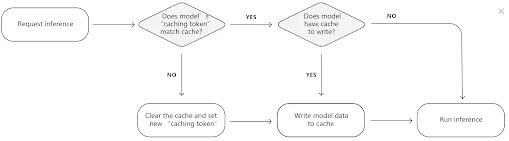cache

Edge TPU 会为每一个模型分配一个缓存令牌，如果当前的模型与缓存令牌一致，则不用更改 cache 中的数据，如果当前模型与缓存令牌不致，则需要重新加载 cache，这样做的好处时，当一个模型长时间运行时，只需要第一次写入缓存即可，加快后续的模型推理速度。

2. TensorFlow Lite Micro (TFLM) 硬件

• Arduino Nano 33 BLE Sense

• SparkFun Edge

• STM32F746 Discovery kit

• Adafruit TensorFlow Lite for Microcontrollers Kit

• Espressif ESP32-DevKitC

• Espressif ESP-EYE

• Wio Terminal: ATSAMD51

• Himax WE-I Plus EVB Endpoint AI Development Board

• Synopsys DesignWare ARC EM Software Development Platform

3. TensorFlow Lite Micro (TFLM) 软件

3.1 算子

3.2 实例

``````# MODEL_TFLITE: TensorFlow Lite模型的路径， *.tflite格式的文件
# MODEL_TFLITE_MICRO: TFLM模型的路径，*.cc格式的文件
# 安装xxd工具
apt-get update && apt-get -qq install xxd
# 把TensorFlow Lite文件转换成c的源文件
xxd -i {MODEL_TFLITE} > {MODEL_TFLITE_MICRO}
# 更新变量名
REPLACE_TEXT = MODEL_TFLITE.replace('/', '_').replace('.', '_')
!sed -i 's/'{REPLACE_TEXT}'/g_model/g' {MODEL_TFLITE_MICRO}
``````

model.pb Keras SavedModel TensorFlow 云/服务器
model.tflite (2.5 kB) TensorFlow Lite 模型，做过整型量化 TensorFlow Lite 移动/嵌入式设备
model.cc C 源码 TFLM 微处理器

1. 加载头文件

``````// 提供运行模型所需要的算子
#include "tensorflow/lite/micro/all_ops_resolver.h"
// 输出调试信息
#include "tensorflow/lite/micro/micro_error_reporter.h"
// 加载并且运行模型
#include "tensorflow/lite/micro/micro_interpreter.h"
// TensorFlow Lite Flatbuffer模型的格式文件的架构
#include "tensorflow/lite/schema/schema_generated.h"
//  TensroFlow Lite的版本信息
#include "tensorflow/lite/version.h"
``````
``````// 需要在整个C代码中，包含以下测试框架
TF_LITE_MICRO_TESTS_BEGIN

.
}

TF_LITE_MICRO_TESTS_END
``````

2. 加载模型

``````const tflite::Model* model = ::tflite::GetModel(g_model);
``````

3.声明解析器

``````tflite::AllOpsResolver resolver;
``````

4. 分配内存 内存大小取决于实际的模型大小，我们也可以使用 `arena_used_bytes` 方法来获取真实的模型大小，用于后续分配相应的内存。

``````const int tensor_arena_size = 2 * 1024;uint8_t tensor_arena[tensor_arena_size];
``````

5. 实例化解析器

``````tflite::MicroInterpreter interpreter(model, resolver, tensor_arena,
tensor_arena_size, error_reporter);
``````

6. 为张量分配内存

``````interpreter.AllocateTensors();
``````

7. 验证张量形状

``````// 首先拿到指向张量的指针，0表示第一个，也是唯一一个输入张量。
TfLiteTensor* input = interpreter.input(0);

// 检查当前的张量形状是我们所预期的
// dims方法表示张量的形状。每个维度都有一个元素，在这个例子中，我们的输入是2D的张量，包括一个元素，所以dims的值应该为2
TF_LITE_MICRO_EXPECT_EQ(2, input->dims->size);
// 每个元素的值表示相应张量的长度，我们这个例子中包括两个单元素张量。
TF_LITE_MICRO_EXPECT_EQ(1, input->dims->data);
TF_LITE_MICRO_EXPECT_EQ(1, input->dims->data);
// 输入的类型是32位的浮点值
TF_LITE_MICRO_EXPECT_EQ(kTfLiteFloat32, input->type);

// KTfLiteFloat32 表示值的类型，完整的列表如下：https://github.com/tensorflow/tflite-micro/blob/main/tensorflow/lite/c/common.h
``````

8. 提供输入

``````input->data.f = 0.;
``````

9. 运行模型

``````// 调用invoke方法运行模型
TfLiteStatus invoke_status = interpreter.Invoke();
if (invoke_status != kTfLiteOk) {
TF_LITE_REPORT_ERROR(error_reporter, "Invoke failed\n");
}
// 断言
TF_LITE_MICRO_EXPECT_EQ(kTfLiteOk, invoke_status);
``````

10. 获取输出

``````TfLiteTensor* output = interpreter.output(0);
TF_LITE_MICRO_EXPECT_EQ(2, output->dims->size);
TF_LITE_MICRO_EXPECT_EQ(1, input->dims->data);
TF_LITE_MICRO_EXPECT_EQ(1, input->dims->data);
TF_LITE_MICRO_EXPECT_EQ(kTfLiteFloat32, output->type);

// 获取张量的输出
float value = output->data.f;
// 在示例代码中，判断值是否在0.05之内
TF_LITE_MICRO_EXPECT_NEAR(0., value, 0.05);
``````

11. 再次运行推理

``````input->data.f = 1.;
interpreter.Invoke();
value = output->data.f;
TF_LITE_MICRO_EXPECT_NEAR(0.841, value, 0.05);

input->data.f = 3.;
interpreter.Invoke();
value = output->data.f;
TF_LITE_MICRO_EXPECT_NEAR(0.141, value, 0.05);

input->data.f = 5.;
interpreter.Invoke();
value = output->data.f;
TF_LITE_MICRO_EXPECT_NEAR(-0.959, value, 0.05);
``````

3.3 编译模型

1. 创建项目

``````make -f tensorflow/lite/micro/tools/make/Makefile \
TARGET=arc_emsdp \
OPTIMIZED_KERNEL_DIR=arc_mli \
generate_hello_world_make_project
``````

2. 编译

``````cd tensorflow/lite/micro/tools/make/gen/arc_emsdp_arc_default/prj/hello_world/make
make app
make flash
``````